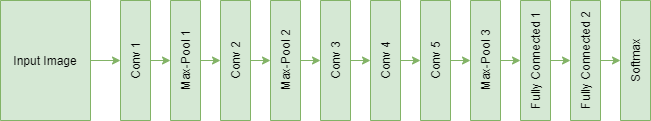# ML | Getting Started With AlexNet

• Last Updated : 26 Mar, 2020

This article is focused on providing an introduction to the AlexNet architecture. Its name comes from one of the leading authors of the AlexNet paper– Alex Krizhevsky. It won the ImageNet Large Scale Visual Recognition Challenge (ILSVRC) 2012 with a top-5 error rate of 15.3% (beating the runner up which had a top-5 error rate of 26.2%).

The most important features of the AlexNet paper are:

• As the model had to train 60 million parameters (which is quite a lot), it was prone to overfitting. According to the paper, the usage of Dropout and Data Augmentation significantly helped in reducing overfitting. The first and second fully connected layers in the architecture thus used a dropout of 0.5 for the purpose. Artificially increasing the number of images through data augmentation helped in the expansion of the dataset dynamically during runtime, which helped the model generalize better.
• Another distinct factor was using the ReLU activation function instead of tanh or sigmoid, which resulted in faster training times (a decrease in training time by 6 times). Deep Learning Networks usually employ ReLU non-linearity to achieve faster training times as the others start saturating when they hit higher activation values.

### The Architecture

The architecture consists of 5 Convolutional layers, with the 1st, 2nd and 5th having Max-Pooling layers for proper feature extraction. The Max-Pooling layers are overlapped having strides of 2 with filter size 3×3. This resulted in decreasing the top-1 and top-5 error rates by 0.4% and 0.3% respectively in comparison to non-overlapped Max-Pooling layers. They are followed by 2 fully-connected layers (each with dropout) and a softmax layer at the end for predictions.

The figure below shows the architecture of AlexNet with all the layers defined.Code: Python code to implement AlexNet for object classification

 `model ``=` `Sequential()`` ` `# 1st Convolutional Layer``model.add(Conv2D(filters ``=` `96``, input_shape ``=` `(``224``, ``224``, ``3``), ``            ``kernel_size ``=` `(``11``, ``11``), strides ``=` `(``4``, ``4``), ``            ``padding ``=` `'valid'``))``model.add(Activation(``'relu'``))``# Max-Pooling ``model.add(MaxPooling2D(pool_size ``=` `(``2``, ``2``),``            ``strides ``=` `(``2``, ``2``), padding ``=` `'valid'``))``# Batch Normalisation``model.add(BatchNormalization())`` ` `# 2nd Convolutional Layer``model.add(Conv2D(filters ``=` `256``, kernel_size ``=` `(``11``, ``11``), ``            ``strides ``=` `(``1``, ``1``), padding ``=` `'valid'``))``model.add(Activation(``'relu'``))``# Max-Pooling``model.add(MaxPooling2D(pool_size ``=` `(``2``, ``2``), strides ``=` `(``2``, ``2``), ``            ``padding ``=` `'valid'``))``# Batch Normalisation``model.add(BatchNormalization())`` ` `# 3rd Convolutional Layer``model.add(Conv2D(filters ``=` `384``, kernel_size ``=` `(``3``, ``3``), ``            ``strides ``=` `(``1``, ``1``), padding ``=` `'valid'``))``model.add(Activation(``'relu'``))``# Batch Normalisation``model.add(BatchNormalization())`` ` `# 4th Convolutional Layer``model.add(Conv2D(filters ``=` `384``, kernel_size ``=` `(``3``, ``3``), ``            ``strides ``=` `(``1``, ``1``), padding ``=` `'valid'``))``model.add(Activation(``'relu'``))``# Batch Normalisation``model.add(BatchNormalization())`` ` `# 5th Convolutional Layer``model.add(Conv2D(filters ``=` `256``, kernel_size ``=` `(``3``, ``3``), ``            ``strides ``=` `(``1``, ``1``), padding ``=` `'valid'``))``model.add(Activation(``'relu'``))``# Max-Pooling``model.add(MaxPooling2D(pool_size ``=` `(``2``, ``2``), strides ``=` `(``2``, ``2``), ``            ``padding ``=` `'valid'``))``# Batch Normalisation``model.add(BatchNormalization())`` ` `# Flattening``model.add(Flatten())`` ` `# 1st Dense Layer``model.add(Dense(``4096``, input_shape ``=` `(``224``*``224``*``3``, )))``model.add(Activation(``'relu'``))``# Add Dropout to prevent overfitting``model.add(Dropout(``0.4``))``# Batch Normalisation``model.add(BatchNormalization())`` ` `# 2nd Dense Layer``model.add(Dense(``4096``))``model.add(Activation(``'relu'``))``# Add Dropout``model.add(Dropout(``0.4``))``# Batch Normalisation``model.add(BatchNormalization())`` ` `# Output Softmax Layer``model.add(Dense(num_classes))``model.add(Activation(``'softmax'``))`

My Personal Notes arrow_drop_up